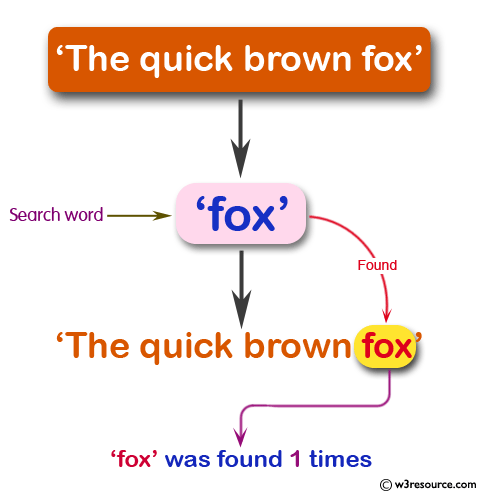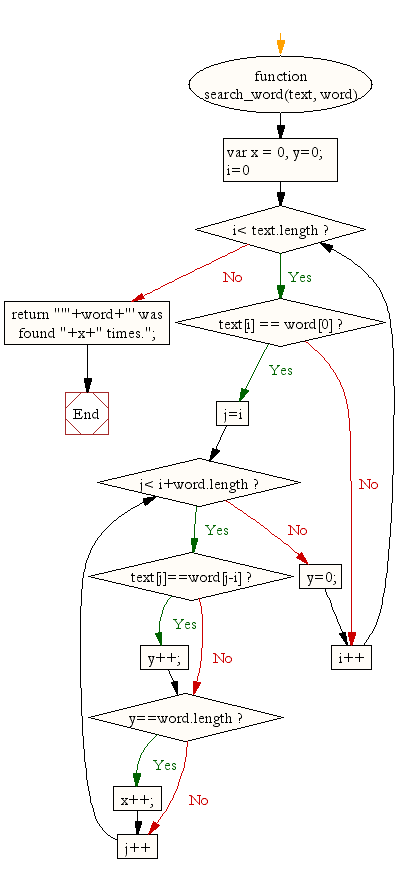# JavaScript: Find a word within a string

## JavaScript String: Exercise-29 with Solution

Write a JavaScript function to find a word within a string.

Test Data:
console.log(search_word('The quick brown fox', 'fox'));
console.log(search_word('aa, bb, cc, dd, aa', 'aa'));
Output:
"'fox' was found 1 times."
"'aa' was found 2 times."

Pictorial Presentation:Sample Solution:-

HTML Code:

``````<!DOCTYPE html>
<html>
<meta charset="utf-8">
<title>JavaScript function to find a word within a string</title>
<body>

</body>
</html>
```
```

JavaScript Code:

``````function search_word(text, word){

var x = 0, y=0;

for (i=0;i< text.length;i++)
{
if(text[i] == word)
{
for(j=i;j< i+word.length;j++)
{
if(text[j]==word[j-i])
{
y++;
}
if (y==word.length){
x++;
}
}
y=0;
}
}
return "'"+word+"' was found "+x+" times.";
}

console.log(search_word('The quick brown fox', 'fox'));
console.log(search_word('aa, bb, cc, dd, aa', 'aa'));
```
```

Sample Output:

```'fox' was found 1 times.
'aa' was found 2 times.
```

Flowchart:Live Demo:

See the Pen JavaScript Find a word within a string - string-ex-29 by w3resource (@w3resource) on CodePen.

Improve this sample solution and post your code through Disqus

What is the difficulty level of this exercise?

﻿

## JavaScript: Tips of the Day

JavaScript: Implicit type coercion

```function sum(a, b) {
return a + b;
}

sum(1, '2');
```

JavaScript is a dynamically typed language: we don't specify what types certain variables are. Values can automatically be converted into another type without you knowing, which is called implicit type coercion. Coercion is converting from one type into another.

In this example, JavaScript converts the number 1 into a string, in order for the function to make sense and return a value. During the addition of a numeric type (1) and a string type ('2'), the number is treated as a string. We can concatenate strings like "Hello" + "World", so what's happening here is "1" + "2" which returns "12".

Ref: https://bit.ly/323Y0P6